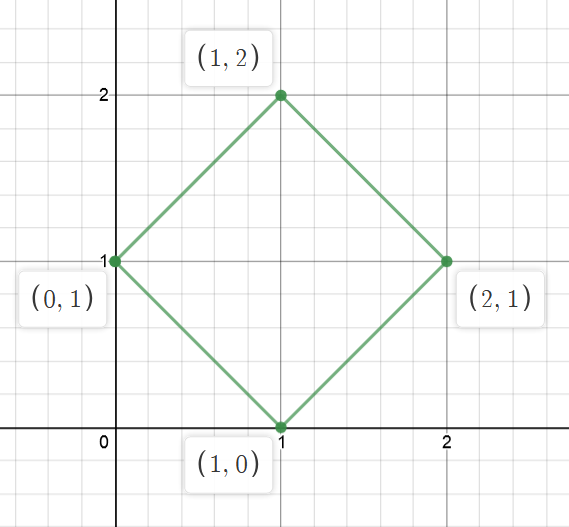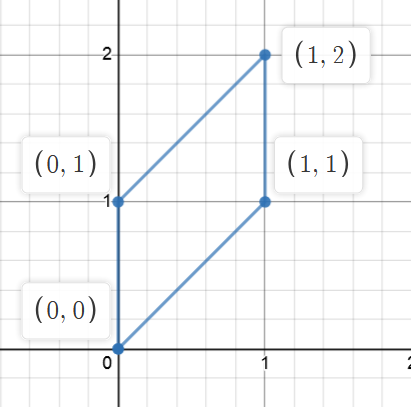Problem title
Difficulty
Avg time to solve

Distinct Paths
Moderate
20 mins
Sorting Of A Rotated Sorted Array
Easy
10 mins
Longest Common Subsequence
Moderate
--
Sub-Matrix with Sum Zero
Moderate
35 mins
Maximum Length of Chain
Moderate
15 mins
3 Divisors
Moderate
25 mins
Angle Between Hour Hand And Minute Hand
Easy
15 mins
Remove maximum edges
Easy
15 mins
Sorted Subsequence of Size 3
Moderate
15 mins
Check Square
Moderate
10 mins2

# Check Square

Difficulty: MEDIUM
Avg. time to solve
10 min
Success Rate
90%

Problem Statement

#### Can you check if those four points make a square?

##### Example:
``````Let the input be [1,0,2,1] and [0,1,1,2].
So, the coordinates of the four points be [ {1, 0}, {0, 1}, {2, 1}, {1, 2} ]
````````````From the above image, we can see that it is a square. Thus, the output will be ‘Yes’.
``````
##### Input format:
``````The first line of input contains an integer ‘T’ denoting the number of test cases.

The first line of each test case contains four space-separated integers representing x-coordinates of the four points.

The second line of each test case contains four space-separated integers representing y-coordinates of the four points.
``````
##### Output format :
``````For each test case, print ‘Yes’ if four points make a square otherwise print ‘No’.
``````
##### Note:
``````Don’t print anything, just return True if four points make a square otherwise return False.
``````
##### Constraints:
``````1 <= T <= 10^4
-10^9 <= xi, yi <= 10^9

Time limit: 1 sec
``````
##### Sample Input 1:
``````2
1 0 2 1
0 1 1 2
1 0 0 1
1 0 1 2
``````
##### Sample Output 1:
``````Yes
No
``````
##### Explanation For Sample Input 1:
``````Test Case 1: Refer to the example described above.

Test Case 2:
The quadrilateral for the given four points is represented below.
````````````As we can clearly see this is not a square. Thus, the answer will be ‘No’.
``````
##### Sample Input 2:
``````2
1 2 4 2
0 2 4 2
0 1 2 3
1 -1 2 0
``````
##### Sample Output 2:
``````No
Yes
``````Console